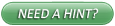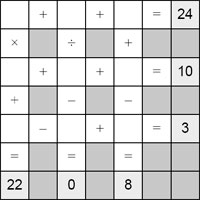## Printable Mathdoku PuzzlesMathdoku puzzles are a mix between a math puzzle and a Sudoku puzzle. You must enter the numbers 1 through 9 into each empty white square, using each number exactly once, so that all six mathematical equations on the grid are fulfilled.

Each puzzle has one and only one unique solution, and most puzzles can be solved using only pure logical deduction. (Trial-and-error methods may occasionally be required for the hardest "Diabolical" puzzles.)## Very Easy Mathdoku## Moderate Mathdoku## Challenging Mathdoku## Diabolical Mathdoku